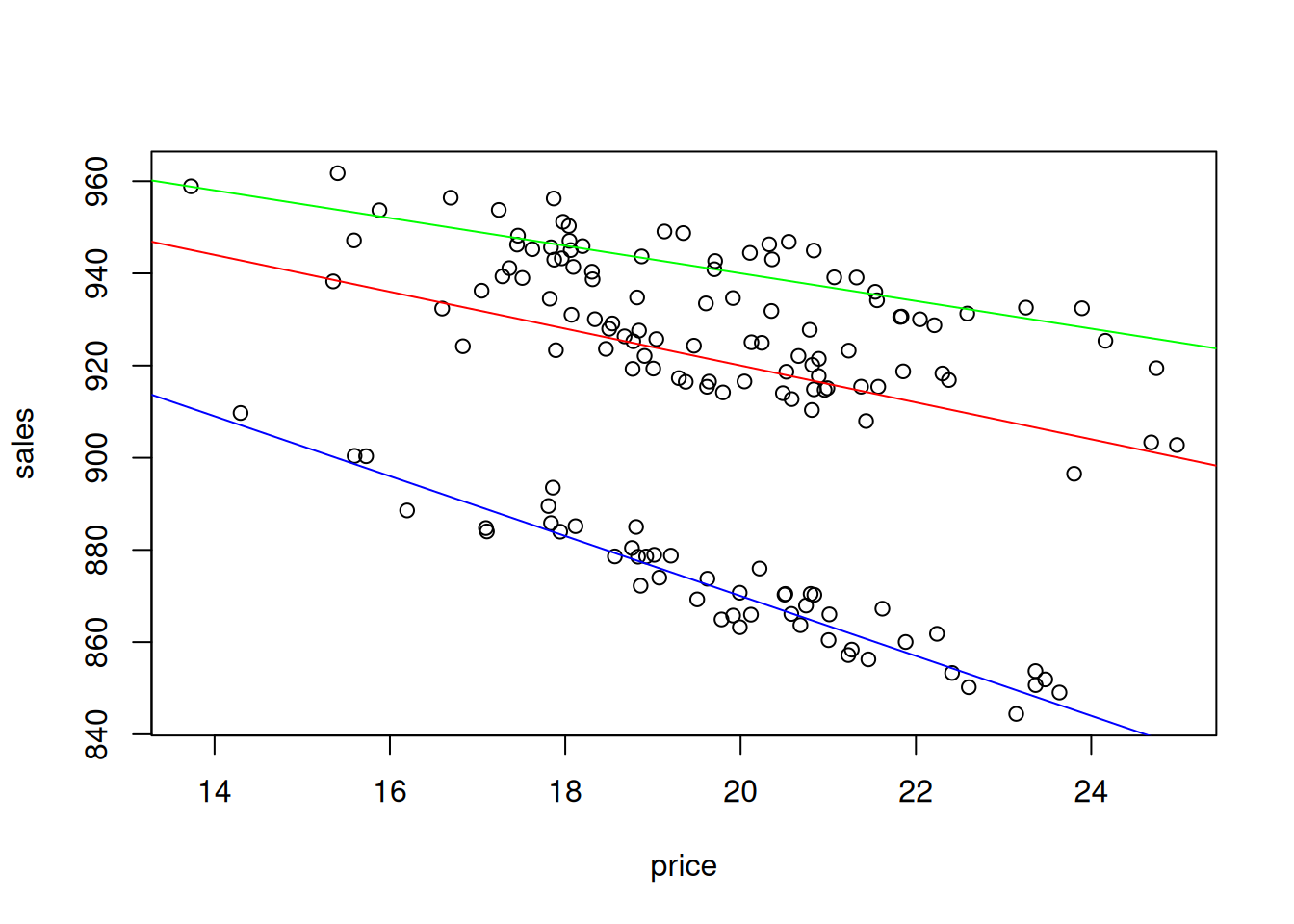This book is in Open Review. I want your feedback to make the book better for you and other readers. To add your annotation, select some text and then click the on the pop-up menu. To see the annotations of others, click the button in the upper right hand corner of the page

## 13.2 Categorical variables for the slope

In reality, we can have more complicated situations, when the change of price would lead to different changes in sales for different types of t-shirts. In this case, we are talking about an interaction effect between price and colour. The following artificial example demonstrates the situation:

tShirtsInteraction <- cbind(rnorm(150,20,2),0,0,0)
tShirtsInteraction[1:50,2] <- tShirtsInteraction[1:50,1]
tShirtsInteraction[1:50+50,3] <- tShirtsInteraction[1:50+50,1]
tShirtsInteraction[1:50+50*2,4] <- tShirtsInteraction[1:50+50*2,1]
tShirtsInteraction <- cbind(1000 + tShirtsInteraction %*% c(-2.5, -1.5, -0.5, -4) +
rnorm(150,0,5), tShirtsInteraction)
colnames(tShirtsInteraction) <- c("sales","price","price:colourRed",
"price:colourGreen","price:colourBlue")

This artificial data can be plotted in the following way to show the effect:

plot(tShirtsInteraction[,2:1])
abline(a=1000, b=-2.5-1.5, col="red")
abline(a=1000, b=-2.5-0.5, col="green")
abline(a=1000, b=-2.5-4, col="blue")Figure 13.3: Scatterplot of Sales vs Price of t-shirts of different colour, interaction effect.

The plot on Figure 13.3 shows that there are three categories of data and that for each of it, the price effect will be different: the increase in price by one unit leads to the faster reduction of sales for the blue t-shirts than for the others. Compare this with Figure 13.2, where we had the difference only in intercepts. This implies a different model: $\begin{equation} sales_j = \beta_0 + \beta_1 price_j + \beta_2 price_j \times colourRed_j + \beta_3 price_j \times colourGreen_j + \epsilon_j . \tag{13.3} \end{equation}$ Notice that we still include only two dummy variables out of three in order to avoid the dummy variables trap. What is new in this case is the multiplication of price by the dummy variables. This trick allows changing the slope of price, depending on the colour of t-shirt. For example, here what the model (13.3) would look like for the three types of colours:

1. Red colour: $$sales_j = \beta_0 + \beta_1 price_j + \beta_2 price_j + \epsilon_j$$ or $$sales_j = \beta_0 + (\beta_1 + \beta_2) price_j + \epsilon_j$$;
2. Green colour: $$sales_j = \beta_0 + \beta_1 price_j + \beta_3 price_j + \epsilon_j$$ or $$sales_j = \beta_0 + (\beta_1 + \beta_3) price_j + \epsilon_j$$;
3. Blue colour: $$sales_j = \beta_0 + \beta_1 price_j + \epsilon_j$$.

In R, the interaction effect can be introduced explicitly in the formula via : symbol if you have a proper factor variable:

tShirtsInteractionDataFrame <- as.data.frame(tShirtsInteraction[,1:2])
tShirtsInteractionDataFrame$colour <- tShirtsDataFrame$colour
# Fit the model
tShirtsInteractionDataFrameALM <- alm(sales~price+price:colour,
tShirtsInteractionDataFrame, loss="MSE")
summary(tShirtsInteractionDataFrameALM)
## Response variable: sales
## Distribution used in the estimation: Normal
## Loss function used in estimation: MSE
## Coefficients:
##                    Estimate Std. Error Lower 2.5% Upper 97.5%
## (Intercept)       1000.4951     4.5501   991.5025   1009.4877 *
## price               -6.5345     0.2304    -6.9900     -6.0791 *
## price:colourGreen    3.5241     0.0503     3.4247      3.6235 *
## price:colourRed      2.4998     0.0498     2.4013      2.5982 *
##
## Error standard deviation: 4.9576
## Sample size: 150
## Number of estimated parameters: 4
## Number of degrees of freedom: 146
## Information criteria:
##      AIC     AICc      BIC     BICc
## 909.9033 910.1791 921.9458 922.6369

Note that the interpretation of parameters in such model will be different, because now the price shows the baseline effect for the blue t-shirts, while the interaction effects show how this effect will change for other colours. So, for example, in order to see what would be the effect of price change on sales of red t-shirts, we need to sum up the parameter for price and price:colourRed. We then can say that if price of red t-shirt increases by £1, the sales will decrease on average by 4.03 units.